Collections of Pauli Operators¶

qecc.PauliList: Sequence type for Pauli operators¶

For convinenence, the qecc package provides a subclass of list intended for use with Pauli operators. PauliList instances can be created either by converting an existing instance of a sequence type, or by providing the elements of the new PauliList.

>>> import qecc as q
>>> L = ['I', 'X', 'Y', 'Z']
>>> print q.PauliList(L)
PauliList(i^0 I, i^0 X, i^0 Y, i^0 Z)
>>> print q.PauliList('XYZ', 'YZX', 'ZXY')
PauliList(i^0 XYZ, i^0 YZX, i^0 ZXY)

Tensor products of a qecc.Pauli` with a PauliList result in tensoring the given Pauli group element onto each element of the list.

>>> from qecc import X
>>> print q.PauliList(L) & X
PauliList(i^0 IX, i^0 XX, i^0 YX, i^0 ZX)

In general, a qecc.PauliList can be used anywhere that a list of qecc.Pauli instances is appropriate. For example, the constructor of qecc.Clifford accepts qecc.PauliList instances:

>>> import qecc as q
>>> C = q.Clifford(q.PauliList('XX', q.Unspecified), q.PauliList(q.Unspecified, q.Pauli('ZZ', phase=2)))
>>> print C
XI |->  +XX
IX |-> Unspecified
ZI |-> Unspecified
IZ |->  -ZZ

Class Reference¶

class qecc.PauliList(*paulis)[source]

Subclass of list offering useful methods for lists of qecc.Pauli instances.

Parameters: paulis – Instances either of str or qecc.Pauli, or the special object qecc.Unspecified. Strings are passed to the constructor of qecc.Pauli for convinenence.

Takes a PauliList, and returns a new PauliList, appending extra_bits qubits, with stabilizer operators specified by lower_right.

Parameters: pauli_list_in – list of Pauli operators to be padded. extra_bits (int) – Number of extra bits to be appended to the system. lower_right – list of qecc.Pauli operators, acting on extra_bits qubits. list of qecc.Pauli objects.

Example:

>>> import qecc as q
>>> pauli_list = q.PauliList('XXX', 'YIY', 'ZZI')
PauliList(i^0 XXXII, i^0 YIYII, i^0 ZZIII, i^0 IIIIX, i^0 IIIZI)
generated_group(coset_rep=None)[source]

Yields an iterator onto the group generated by this list of Pauli operators. See also qecc.from_generators.

stabilizer_subspace()[source]

Returns a numpy.ndarray of shape (n - k, 2 ** n) containing an orthonormal basis for the mutual +1 eigenspace of each fully specified Pauli in this list. Here, n is taken to be the number of qubits and k is taken to be the number of independent Pauli operators in this list.

Raises a RuntimeError if NumPy cannot be imported.

For example, to find the Bell basis vector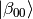using the stabilizer formalism:

>>> import qecc as q
>>> q.PauliList('XX', q.Unspecified, q.Unspecified, 'ZZ').stabilizer_subspace()
array([[ 0.70710678+0.j,  0.00000000+0.j,  0.00000000+0.j,  0.70710678+0.j]])

Similarly, one can find the codewords of the phase-flip code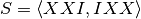:

>>> q.PauliList('XXI', 'IXX').stabilizer_subspace()
array([[ 0.50000000+0.j,  0.00000000-0.j,  0.00000000-0.j,  0.50000000+0.j,
0.00000000-0.j,  0.50000000+0.j,  0.50000000+0.j,  0.00000000-0.j],
[ 0.02229922+0.j,  0.49950250+0.j,  0.49950250+0.j,  0.02229922+0.j,
0.49950250+0.j,  0.02229922+0.j,  0.02229922+0.j,  0.49950250+0.j]])

Note that in this second case, some numerical errors have occured; this method does not guarantee that the returned basis vectors are exact.

centralizer_gens(group_gens=None)[source]

Returns the generators of the centralizer group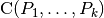, where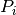is the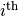element of this list. See qecc.Pauli.centralizer_gens() for more information.